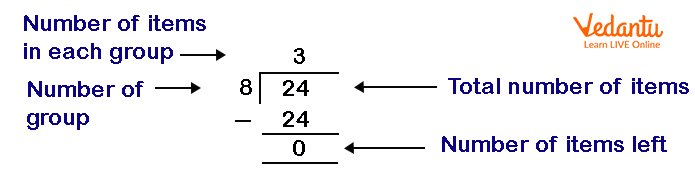Courses
Courses for Kids
Free study material
Free LIVE classes
More

# Partitive Division Word ProblemsLIVE
Join Vedantu’s FREE Mastercalss

## Introduction To Division Problem.

Generally, there are two ways to think about division problems. The two ways are partitive division and Measurement Division.

A partitive division, also known as partition, sharing, and grouping division, is a division problem in which you divide an item into a given number of groups.

For example, 25 5, states that there are 25 things that are evenly divided among 5 groups, and we need to know how many things are there in one or each group.

A measurement division, also known as repeated subtraction division, is a division problem in which you divide an item into a group of given size.

For example, 25 5, states that 25 things are evenly divided into groups of 5, and we need to know how many total groups we can make.

Read below to know what partitive divisions are?

## What Is Partitive Division?

A partitive division is a division problem where you know the total number of groups, and you need to find the number of items in each group.

For example, There are 18 apples equally distributed in 6 groups. How many apples are there in each basket?

## How To Solve Partitive Division Word Problems?

In Partitive division, we can find the number of items in each group using two ways. The two ways are:

### 1. Using Repeated Subtraction:

In repeated subtraction, we first subtract the total number of groups from the total number of iems. Then, we subtract the number of groups from the result obtained in the previous step. We keep on subtracting the number until we find the remainder 0 or left with the number smaller than the number of groups.

The total number of times we subtract the number of groups gives the number of items in each group.

Let us understand with an example,

Example:

Suppose, there are 24 cookies equally distributed in 8 groups. Find how many cookies are there in each basket using repeated subtraction.

Solution:

Number of Jars : 8

According to the repeated subtraction, we will first first subtract the total number of cookies from the number of jars we have. Accordingly, we get

24 - 8 = 16

Now, we will keep on subtracting the number of jars from the result obtained in the previous step till we get the remainder 0, or the number less than number of jars i.e.8. Accordingly, we get

16 - 8 = 8

8 - 8 = 0

Here, we have subtracted the number of jars i.e. 8 three times. Hence, the number of cookies in each jar is 3.

### 2. Using Division:

To find the number of items in each group using division, we can simply divide the total number of items (dividend) by the total number of groups (divisor). The quotient obtained is considered as the number of items in each group.

Let us understand with an example:

Suppose, there are 24 apples equally distributed in 6 baskets. Find how many apples are there in each basket using division.

Solution:

Total Number of Apples: 24

Number of apples in each basket: 24 8Using Division

Therefore, the number of apples in each basket is 3.

Last updated date: 21st Sep 2023
Total views: 134.4k
Views today: 1.34k